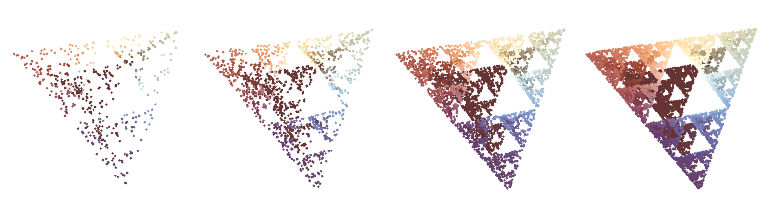I recently encountered this episode of Engines of Our Ingenuity, where they describe the following “game”:

We start by picking any point in the triangle and drawing a dot. Let’s call it dot 1. We pick the next dot as follows. Randomly choose one of the triangle’s three corners, and draw a dot midway between that corner and dot 1. Then just iterate; that is, randomly pick another corner of the triangle and draw a point that’s midway between that corner and dot 2. Call it dot 3. And repeat.

It’s a very simple process. But what emerges is stunning.

What emerges is the Sierpinski Gasket; what’s surprising is that this seemingly arbitrary procedure tends toward such an intricate structure. I couldn’t visualize what was happening at first, nor did my freehand attempts seem to be converging to the expected result. So, I wrote a short Processing program to visualize it:

``````ArrayList<PVector> triangle = new ArrayList<PVector>();
PVector p0;
PVector lastPoint;

void setup() {
size(800, 700);
smooth();
background(225);
}

void draw() {
// draw the initial triangle in red
stroke(200, 0, 0);
strokeWeight(4);
for (int i = 0; i < triangle.size(); i++) {
point(triangle.get(i).x, triangle.get(i).y);
}

// draw the bounds of the triangle (so far)
strokeWeight(2);
for (int i = 1; i <= triangle.size(); i++) {
line(triangle.get(i-1).x, triangle.get(i-1).y, triangle.get(i % triangle.size()).x, triangle.get(i % triangle.size()).y);
}

if (p0 != null) {
// draw the initial point in red also
point(p0.x, p0.y);

// draw every following point smaller, in black
stroke(0, 0, 0);
strokeWeight(1.25);

final int POINTS_PER_FRAME = 10;
for (i = 0; i < POINTS_PER_FRAME; i++) {
// pick a random vertex of the bounding triangle
float r = random(0, 2.999999);
int randomIndex = int(r);
PVector randomVertex = triangle.get(randomIndex);

// find midpoint between this and last point:
// this way would be nice,
//   PVector newPoint = lastPoint.lerp(randomVertex, 0.5);
// but this way appears to be necessary for processing.js
PVector newPoint = new PVector(lerp(randomVertex.x, lastPoint.x, 0.5), lerp(randomVertex.y, lastPoint.y, 0.5));
point(newPoint.x, newPoint.y);

lastPoint = newPoint;
}
}
}

void mouseClicked() {
if (triangle.size() < 3) {
} else if (p0 == null) {
p0 = new PVector(mouseX, mouseY);
lastPoint = p0;
}
}

void keyPressed() {
if (key=='r' || key == 'R') {
// reset
background(225);
p0 = null;
triangle.clear();
}
}``````

Click three times to define the triangle, then once more to set the starting point. Then watch the Sierpinski Gasket emerge like a exposed emulsion in a darkroom’s developer tray (type ‘r’ to reset and begin again):

### Update 2/14/2015

I was curious whether this algorithm would extend to three dimensions, so I used the following snippet of Mathematica code to see:

``````vertices = {{1, 1, 1}, {1, -1, -1}, {-1, 1, -1}, {-1, -1, 1}};
pts = {};
startingpt = {0, 0, 0};
Lerp[x_, y_, t_ ] := x + t (y - x);
Do[newpoint = Lerp[startingpt, RandomChoice[vertices], 0.5];
AppendTo[pts, newpoint];
startingpt = newpoint, {i, 1, 50000}];
Graphics3D[Point[pts, VertexNormals -> pts], Boxed -> False]``````It certainly would appear that it does.